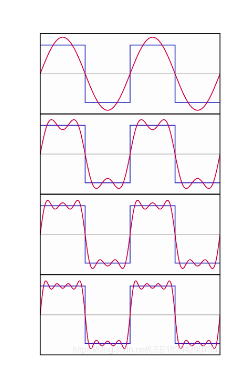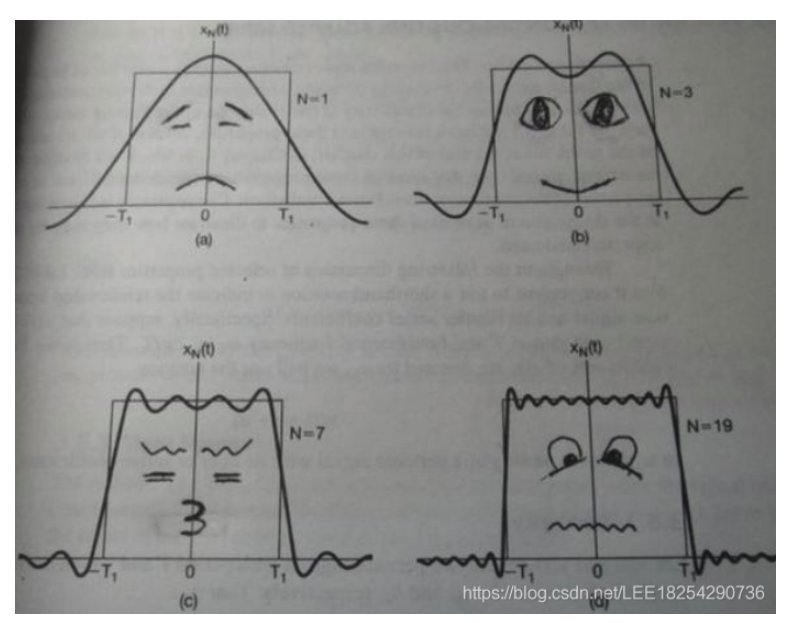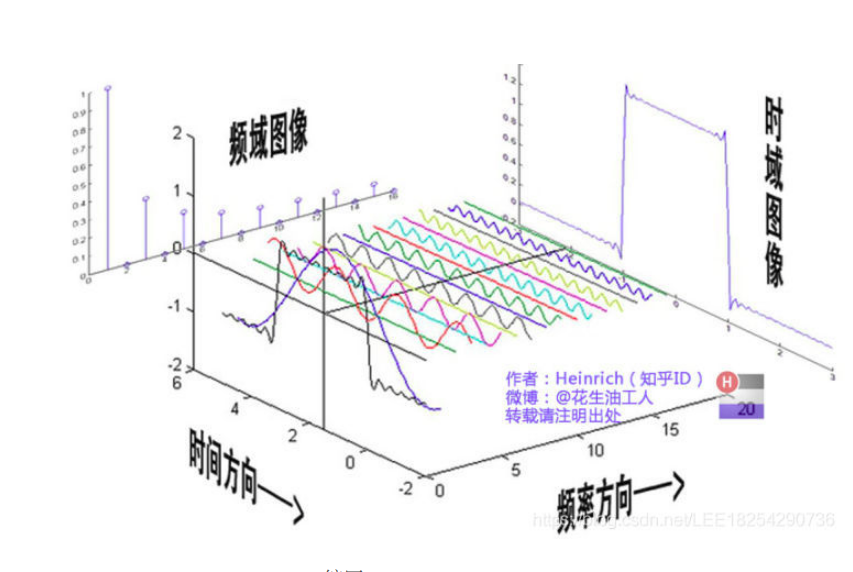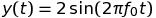• 频域分析法1. 频域分析法定义2. 目的与意义3. 适用范围4. 优缺点4.1优点4.2 缺点5. 分析过程 1. 频域分析法定义 频域分析法是一种研究控制系统的经典方法。是在频域范围内应用图解分析法评价系统性能的一种工程方法...
• MATLAB与频域分析-利用MATLAB实现系统的频域分析.pdf 这个主要是控制工程中频域分析法以MATLAB来实现，绘制BODE图，NYQUIST图更快捷方便matlab
• MATLAB与频域分析-基于MATLAB的系统频域分析的实现.pdf 这个主要是控制工程中频域分析法以MATLAB来实现，绘制BODE图，NYQUIST图更快捷方便matlab
• MATLAB与频域分析-一种基于MATLAB的控制系统频域分析方法.pdf 这个主要是控制工程中频域分析法以MATLAB来实现，绘制BODE图，NYQUIST图更快捷方便matlab
• MATLAB与频域分析-基于Matlab的_信号与系统_频域分析.pdf 这个主要是控制工程中频域分析法以MATLAB来实现，绘制BODE图，NYQUIST图更快捷方便matlab
• MATLAB实验文档：熟悉掌握MATLAB中频域的分析方法用MATLAB进行系统频域分析与设计，帮助您更好的进行频域分析与设计
• 使用Python实现一段信号的频域分析与包络谱分析
• ## 频域分析基础

千次阅读 多人点赞 2019-04-07 21:01:07
频域分析基础 此部分内容为频域分析中一些经典结论的推导过程，用作积累与巩固基础知识。 描述控制系统在不同频率的正弦函数作用时的稳态输出和输入信号之间关系的数学模型称为频率特性，它反映了在正弦信号作用下...
频域分析基础
此部分内容为频域分析中一些经典结论的推导过程，用作积累与巩固基础知识。
描述控制系统在不同频率的正弦函数作用时的稳态输出和输入信号之间关系的数学模型称为频率特性，它反映了在正弦信号作用下系统响应的性能。应用频率特性研究线性系统的经典方法称为频域分析法。在常规的控制理论中，频域响应法往往是最有效的，因为我们可以利用对物理系统实测得到的数据来分析系统性能，而不需要推导出系统的精确的数学模型；而且采用容易提供的正弦信号发生器和精密的测量装置，完成频率响应的实验通常比较简单，并且可以做的很精确。
但是，通常一提到控制系统中的频域分析，大家第一时间都会想到：令传递函数的

s

=

j

w

s=jw

但是，为什么要做这样的变换，以及这个等式背后的原因，却很少有人去关注。
首先，我们来看如下结论：
对于线性时不变系统

G

(

s

)

G(s)

系统的输入量和输出量分别用

x

(

t

)

x(t)

和

y

(

t

)

y(t)

表示,如果输入量为正弦信号，则系统的稳态输出量也是一个相同频率的正弦信号，但是可能有不同的幅值和相角
推导： 我们假设系统的正弦输出为如下形式：

x

(

t

)

=

X

s

i

n

w

t

x(t)=Xsinwt

，其中

w

(

r

a

d

/

s

)

为角频率，且有

w

=

2

π

f

,

f

(

c

i

r

c

l

e

/

s

)

w=2\pi f,f(circle/s)

且设系统的传递函数可写成两个多项式之比的形式，如下

G

(

s

)

=

p

(

s

)

q

(

s

)

=

p

(

s

)

(

s

+

s

1

)

(

s

+

s

2

)

…

(

s

+

s

n

)

G(s)=\frac {p(s)} {q(s)} = \frac {p(s)} {(s+s_1) (s+s_2) …(s+s_n)}

则系统输出的拉普拉斯变化可写成如下形式：

Y

(

s

)

=

G

(

s

)

X

(

s

)

=

p

(

s

)

q

(

s

)

X

(

s

)

Y(s)= G(s)X(s)=\frac {p(s)} {q(s)}X(s)

而系统输入的拉普拉斯变换可以写成如下形式：

X

(

s

)

=

w

X

s

2

+

w

2

X(s)= \frac {wX} {s^2+w^2}

注： 对于更一般的

x

(

t

)

=

X

s

i

n

(

w

t

+

ϕ

i

)

x(t)=Xsin(wt+\phi_i)

的形式，我们作如下变换：

X

s

i

n

(

w

t

+

ϕ

i

)

=

X

(

s

i

n

w

t

c

o

s

ϕ

i

+

c

o

s

w

t

s

i

n

ϕ

i

)

=

A

s

i

n

w

t

+

B

c

o

s

w

t

Xsin(wt+\phi_i)=X(sinwtcos\phi_i+coswtsin\phi_i)=Asinwt+Bcoswt

再做拉普拉斯变换：

A

w

s

2

+

w

2

+

B

s

s

2

+

w

2

\frac{Aw}{s^2+w^2}+\frac{Bs}{s^2+w^2}

再带入频域响应的表达式中即可，只是这样推导过程较为繁琐，故本为以

x

(

t

)

=

X

s

i

n

w

t

x(t)=Xsinwt

为例。
则

Y

(

s

)

=

G

(

s

)

X

(

s

)

=

p

(

s

)

w

X

(

s

2

+

w

2

)

(

s

+

s

1

)

(

s

+

s

2

)

…

(

s

+

s

n

)

Y(s)= G(s)X(s)= \frac {p(s) wX } {( s^2+w^2)(s+s_1) (s+s_2) …(s+s_n)}

部分分式展开可得：

Y

(

s

)

=

a

(

s

+

j

w

)

+

a

ˉ

(

s

−

j

w

)

+

b

1

(

s

+

s

1

)

+

b

2

(

s

+

s

2

)

…

+

b

n

(

s

+

s

n

)

Y(s)= \frac {a} {(s+jw)} +\frac {\bar{a}} {(s-jw)} +\frac {b_1} {(s+s_1)} +\frac {b_2} {(s+s_2)}…+\frac {b_n} {(s+s_n)}

其中

a

ˉ

\bar{a}

是

a

a

的共轭复数，

a

,

b

i

a,b_i

为常数，对上式进行拉普拉斯反变换可得：

y

(

t

)

=

a

e

−

j

w

t

+

a

ˉ

e

j

w

t

+

b

1

e

−

s

1

t

+

b

2

e

−

s

2

t

…

+

b

n

e

−

s

n

t

y(t)=ae^{-jwt}+ \bar{a}e^{jwt}+b_1e^{-s_1t}+b_2e^{-s_2t}…+b_ne^{-s_nt}

对于一个稳态系统，其闭环极点均位于复平面左侧，故

−

s

i

&lt;

0

-s_i&lt;0

,当

t

t

趋近于无穷时，

b

i

e

−

s

i

t

b_ie^{-s_it}

趋近于0，因此系统的稳态响应将变为如下形式：

y

s

s

(

t

)

=

a

e

−

j

w

t

+

a

ˉ

e

j

w

t

y_{ss}(t)= ae^{-jwt}+ \bar{a}e^{jwt}

式中常数可根据留数定理得到：

a

=

G

(

s

)

w

X

s

2

+

w

2

(

s

+

j

w

)

∣

s

=

−

j

w

=

−

X

G

(

−

j

w

)

2

j

a=G(s) \frac { wX } {s^2+w^2} (s+jw)|_{s=-jw}=-\frac {XG(-jw)} {2j}

a

ˉ

=

G

(

s

)

w

X

s

2

+

w

2

(

s

−

j

w

)

∣

s

=

j

w

=

X

G

(

j

w

)

2

j

\bar{a}=G(s) \frac { wX } {s^2+w^2} (s-jw)|_{s=jw}=\frac {XG(jw)} {2j}

上式中，

G

(

j

w

)

G(jw)

是一个复数量，我们作如下定义

G

(

j

w

)

=

∣

G

(

j

w

)

∣

e

j

ϕ

G(jw)=|G(jw)|e^{j\phi}

其中

∣

G

(

j

w

)

∣

|G(jw)|

表示

G

(

j

w

)

G(jw)

的幅值，

ϕ

=

∠

G

(

j

w

)

=

a

r

c

t

a

n

[

G

(

j

w

)

的

虚

部

G

(

j

w

)

的

实

部

]

\phi=\angle{G(jw)}=arctan[\frac { G(jw)的虚部} {G(jw)的实部}]

表示

G

(

j

w

)

G(jw)

的相角，类似的

G

(

−

j

w

)

=

∣

G

(

−

j

w

)

∣

e

−

j

ϕ

=

∣

G

(

j

w

)

∣

e

−

j

ϕ

，

G(-jw)=|G(-jw)|e^{-j\phi}=|G(jw)|e^{-j\phi}，

到此，系统的稳态响应可以写为如下形式：

y

s

s

(

t

)

=

X

∣

G

(

j

w

)

∣

e

j

(

w

t

+

ϕ

)

2

j

−

X

∣

G

(

j

w

)

∣

e

−

j

(

w

t

+

ϕ

)

2

j

y_{ss}(t)= \frac {X|G(jw)|e^{j(wt+\phi)}} {2j} - \frac {X|G(jw)|e^{-j(wt+\phi)}} {2j}

由欧拉公式：

e

j

θ

=

c

o

s

θ

+

j

s

i

n

θ

e^{j\theta}=cos\theta +jsin\theta

上式变为：

y

s

s

(

t

)

=

X

∣

G

(

j

w

)

∣

2

j

[

(

c

o

s

(

w

t

+

ϕ

)

+

j

s

i

n

(

w

t

+

ϕ

)

)

−

(

c

o

s

(

w

t

+

ϕ

)

−

j

s

i

n

(

w

t

+

ϕ

)

)

]

=

X

∣

G

(

j

w

)

∣

s

i

n

(

w

t

+

ϕ

)

y_{ss}(t)= \frac {X|G(jw)|}{2j}[(cos(wt+\phi)+jsin(wt+\phi))-(cos(wt+\phi)-j sin(wt+\phi))] =X|G(jw)|sin(wt+\phi)

输出信号的幅值

Y

=

X

∣

G

(

j

w

)

∣

Y=X|G(jw)|

在此基础上，我们得到如下结论：对于正弦输入信号输出信号的振幅由输入信号的振幅

X

X

与

∣

G

(

j

w

)

∣

|G(jw)|

的乘积给出，而相角则与输入信号相差一个量值

ϕ

=

∠

G

(

j

w

)

\phi=\angle{G(jw)}

因此系统对正弦输入信号的稳态响应特性可直接由下式给出：

G

(

j

w

)

=

Y

(

j

w

)

X

(

j

w

)

G(jw)=\frac{Y(jw)}{X(jw)}

这便是频域分析中直接将

s

=

j

w

s=jw

的原因。
附一段百度百科的介绍：
频域分析法具有以下特点：
首先，控制系统及其元部件的频率特性可以运用分析法和实验方法获得，并可用多种形式的曲线表示，因而系统分析和控制器设计可以应用图解法进行。 其次，频率特性物理意义明确。对于一阶系统和二阶系统，频域性能指标和时域性能指标有确定的对应关系；对于高阶系统，可建立近似的对应关系。 第三，控制系统的频域设计可以兼顾动态响应和噪声抑制两方面的要求。 第四，频域分析法不仅适用于线性定常系统，还可以推广应用于某些非线性控制系统。
频域分析法就是将信号分解为正弦波，并且用正弦波合成信号。分解或分析就是计算各种频率的正弦波在信号中所占的比例，合成或综合就是根据不同比例的正弦波来合成信号。对分解的信号可以如此处理：保留部分幅值比较大的正弦波分量，以备将来恢复信号。这样做在实践中有不少好处，例如减少表示信号所需的数据、节省存储数据的存储器、节省传输数据的时间、增加通信线路的使用效率等。合成信号也有不少用途，例如恢复接收数据的原始信号、合成语音信号、生成图像信号、产生测量信号等。
频域分析法是从频率的角度看问题，它能看到时域角度看不到的问题。频域分析法的优点是：它引导人们从信号的表面深入到信号的本质，看到信号的组成部分。通过对成分的了解，人们可以更好地使用信号。这种做法很像化学分析，比如污水处理，化学分析能够帮助工程师了解污水处理的效果，达到改进和提高处理方法的目的。有了信号分析的概念，就提高了人们的观察力。
展开全文• MATLAB与频域分析-基于MATLAB的语音信号时频域参数分析.pdf 这个主要是控制工程中频域分析法以MATLAB来实现，绘制BODE图，NYQUIST图更快捷方便matlab
• 时域频域分析论文集，时域频域分析论文集，时域频域分析论文集，时域频域分析论文集，时域频域分析论文集，
• 时域分析与频域分析:https://blog.csdn.net/lee18254290736/article/details/50357504/
时域分析与频域分析:https://blog.csdn.net/lee18254290736/article/details/50357504/展开全文• ## 如何使用matlab进行频域分析

万次阅读 多人点赞 2018-08-24 20:17:27
本文讨论使用Matlab对信号进行频域分析的方法。 说到频域，不可避免的会提到傅里叶变换，傅里叶变换提供了一个将信号从时域转变到频域的方法。之所以要有信号的频域分析，是因为很多信号在时域不明显的特征可以在...
Matlab可以说是一个非常有用且功能齐全的工具，在通信、自控、金融等方面有广泛的应用。
本文讨论使用Matlab对信号进行频域分析的方法。
说到频域，不可避免的会提到傅里叶变换，傅里叶变换提供了一个将信号从时域转变到频域的方法。之所以要有信号的频域分析，是因为很多信号在时域不明显的特征可以在频域下得到很好的展现，可以更加容易的进行分析和处理。

FFT
Matlab提供的傅里叶变换的函数是FFT，中文名叫做快速傅里叶变换。快速傅里叶变换的提出是伟大的，使得处理器处理数字信号的能力大大提升，也使我们生活向数字化迈了一大步。
接下来就谈谈如何使用这个函数。
fft使用很简单，但是一般信号都有x和y两个向量，而fft只会处理y向量，所以想让频域分析变得有意义，那么就需要用户自己处理x向量

一个简单的例子
从一个简单正弦信号开始吧，正弦信号定义为：我们现在通过以下代码在Matlab中画出这个正弦曲线
fo = 4; %frequency of the sine wave

Fs = 100; %sampling rate

Ts = 1/Fs; %sampling time interval

t = 0:Ts:1-Ts; %sampling period

n = length(t); %number of samples

y = 2*sin(2*pi*fo*t); %the sine curve

%plot the cosine curve in the time domain

sinePlot = figure;

plot(t,y)

xlabel('time (seconds)')

ylabel('y(t)')

title('Sample Sine Wave')

grid

这就是我们得到的：当我们对这条曲线fft时，我们希望在频域得到以下频谱（基于傅里叶变换理论，我们希望看见一个幅值为1的峰值在-4Hz处，另一个在+4Hz处）使用FFT命令
我们知道目标是什么了，那么现在使用Matlab的内建的FFT函数来重新生成频谱

%plot the frequency spectrum using the MATLAB fft command

matlabFFT = figure; %create a new figure

YfreqDomain = fft(y); %take the fft of our sin wave, y(t)

stem(abs(YfreqDomain)); %use abs command to get the magnitude

%similary, we would use angle command to get the phase plot!

%we'll discuss phase in another post though!

xlabel('Sample Number')

ylabel('Amplitude')

title('Using the Matlab fft command')

grid

axis([0,100,0,120])
效果如下：但是注意一下，这并不是我们真正想要的，有一些信息是缺失的

x轴本来应该给我们提供频率信息，但是你能读出频率吗？  幅度都是100  没有让频谱中心为
为FFT定义一个函数来获取双边频谱
以下代码可以简化获取双边频谱的过程，复制并保存到你的.m文件中
function [X,freq]=centeredFFT(x,Fs)

%this is a custom function that helps in plotting the two-sided spectrum

%x is the signal that is to be transformed

%Fs is the sampling rate

N=length(x);

%this part of the code generates that frequency axis

if mod(N,2)==0

k=-N/2:N/2-1; % N even

else

k=-(N-1)/2:(N-1)/2; % N odd

end

T=N/Fs;

freq=k/T; %the frequency axis

%takes the fft of the signal, and adjusts the amplitude accordingly

X=fft(x)/N; % normalize the data

X=fftshift(X); %shifts the fft data so that it is centered
这个函数输出正确的频域范围和变换后的信号，它需要输入需要变换的信号和采样率。
接下来使用前文的正弦信号做一个简单的示例，注意你的示例.m文件要和centeredFFT.m文件在一个目录下
[YfreqDomain,frequencyRange] = centeredFFT(y,Fs);

centeredFFT = figure;

%remember to take the abs of YfreqDomain to get the magnitude!

stem(frequencyRange,abs(YfreqDomain));

xlabel('Freq (Hz)')

ylabel('Amplitude')

title('Using the centeredFFT function')

grid

axis([-6,6,0,1.5])
效果如下：这张图就满足了我们的需求,我们得到了在+4和-4处的峰值，而且幅值为1.

为FFT定义一个函数来获取右边频谱
从上图可以看出，FFT变换得到的频谱是左右对称的，因此，我们只需要其中一边就能获得信号的所有信息，我们一般保留正频率一侧。
以下的函数对上面的自定义函数做了一些修改，让它可以帮助我们只画出信号的正频率一侧
function [X,freq]=positiveFFT(x,Fs)

N=length(x); %get the number of points

k=0:N-1; %create a vector from 0 to N-1

T=N/Fs; %get the frequency interval

freq=k/T; %create the frequency range

X=fft(x)/N; % normalize the data

%only want the first half of the FFT, since it is redundant

cutOff = ceil(N/2);

%take only the first half of the spectrum

X = X(1:cutOff);

freq = freq(1:cutOff);

和前面一样，使用正弦信号做一个示例，下面是示例代码
[YfreqDomain,frequencyRange] = positiveFFT(y,Fs);

positiveFFT = figure;

stem(frequencyRange,abs(YfreqDomain));

set(positiveFFT,'Position',[500,500,500,300])

xlabel('Freq (Hz)')

ylabel('Amplitude')

title('Using the positiveFFT function')

grid

axis([0,20,0,1.5])
效果如下：--------------------------------------------
本文内容主要翻译自Matlab官方FFT教程，但是我找不到原链接了，就把我电脑上的文件上传到了百度云盘，公众号后台回复
下载|FFT_tutorial
获取下载链接
------------------------------------------------
本文代码以上传github，可自行下载测试，链接：
https://github.com/greedyhao/DSP/tree/master/matlab_tutorial
-------------------------------------------------展开全文matlab
• 随机信号分析，包含对于随机信号的时域频域分析，相关性分析，高斯白噪声分析matlab
• matlab程序中，标准的频域分析，包含一个信号例子
• 是德科技有限公司日前宣布推出频域分析选件（FDA），这是业界适用于实时示波器的用户可扩展的频谱/频域分析解决方案。 　选件FDA可显着增进Keysight Infiniium和InfiniiVision系列示波器的功能。利用该选件，工程师...
• MATLAB 电子信息应用 课程设计 设计五 信号的频域分析及 MATLAB 实现 学院 专业 班级 姓名 学号 信号的频域分析及 MATLAB 实现 一 设计目的 通过该设计理解傅里叶变换的定义及含义掌握对 信号进行频域分析的方法 二 ...
• labwindows/CVI声音时域频域分析，并判定频率参数进行判定报警
• MatLab时域分析与频域分析的程序，已经运行成功，而且比较准确，有兴趣的人可以拿来使用。 MatLab时域分析与频域分析的程序，已经运行成功，而且比较准确，有兴趣的人可以拿来使用。
• 频域分析法—自控学习ppt,频域分析法—自控学习
•Simulink基础
• 自动控制原理频域分析法课件，适合大学生的自学以及考试
• 离散周期信号的频域分析（DFS），非周期信号的频域分析（DTFT），离散傅里叶变换（DFT）...